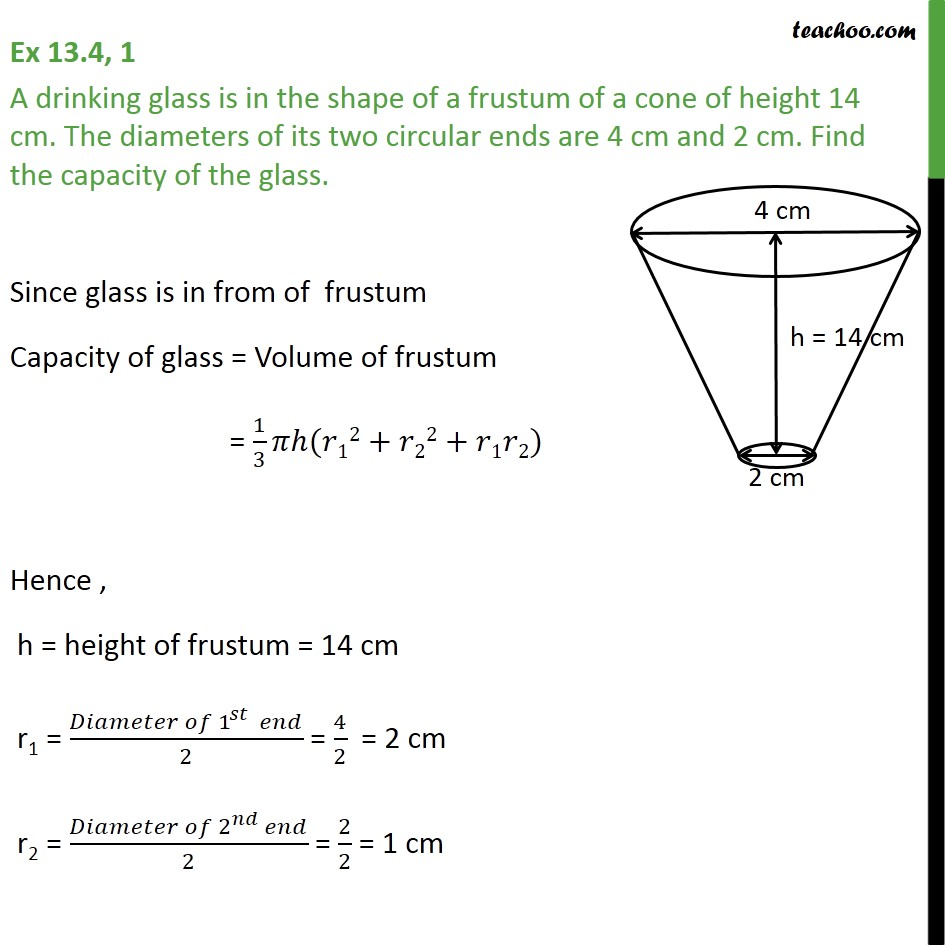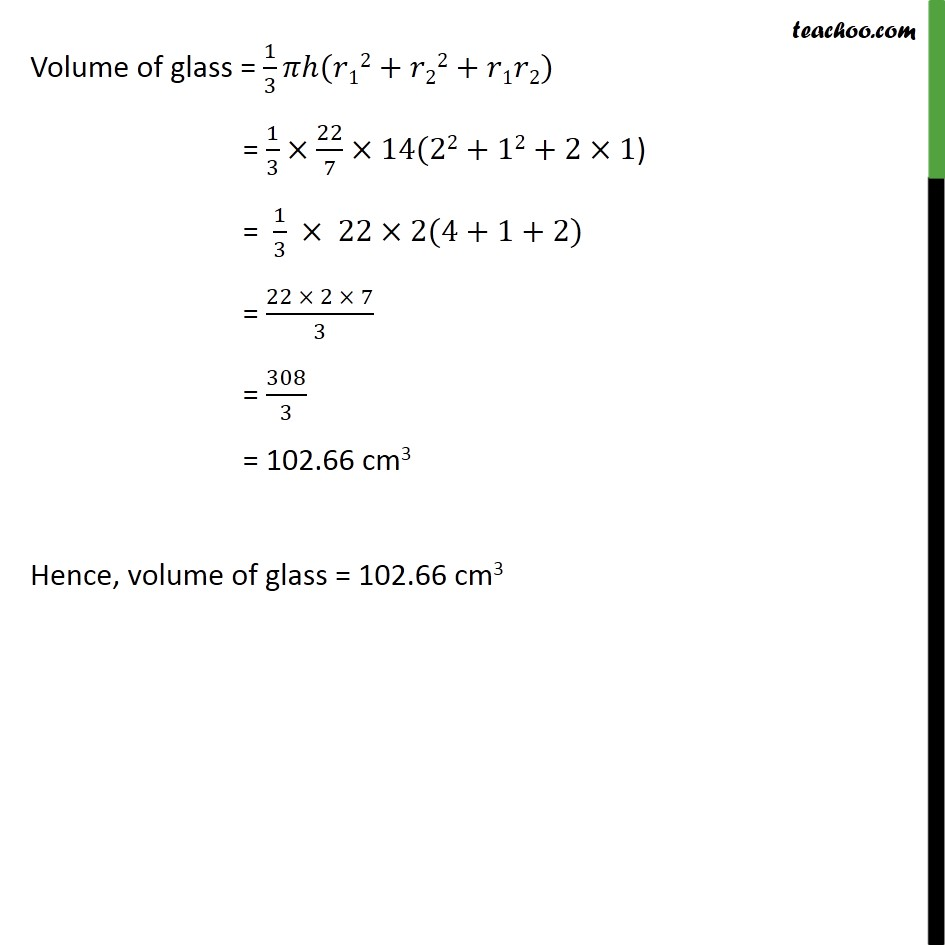Subscribe to our Youtube Channel - https://you.tube/teachoo

1. Chapter 13 Class 10 Surface Areas and Volumes
2. Concept wise
3. Frustum of a cone - Volume

Transcript

Ex 13.4, 1 A drinking glass is in the shape of a frustum of a cone of height 14 cm. The diameters of its two circular ends are 4 cm and 2 cm. Find the capacity of the glass. Since glass is in from of frustum Capacity of glass = Volume of frustum = 1/3 𝜋ℎ(𝑟12+𝑟22+𝑟1𝑟2) Hence , h = height of frustum = 14 cm r1 = (𝐷𝑖𝑎𝑚𝑒𝑡𝑒𝑟 𝑜𝑓 1^𝑠𝑡 𝑒𝑛𝑑)/2 = 4/2 = 2 cm r2 = (𝐷𝑖𝑎𝑚𝑒𝑡𝑒𝑟 𝑜𝑓 2^𝑛𝑑 𝑒𝑛𝑑)/2 = 2/2 = 1 cm Volume of glass = 1/3 𝜋ℎ(𝑟12+𝑟22+𝑟1𝑟2) = 1/3×22/7×14(22+12+2×1) = 1/3 × 22×2(4+1+2) = (22 × 2 × 7)/3 = 308/3 = 102.66 cm3 Hence, volume of glass = 102.66 cm3

Frustum of a cone - Volume